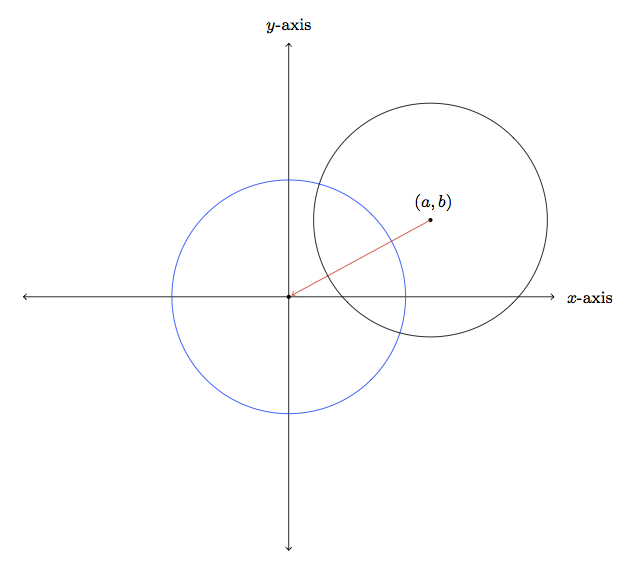# Similar circles

Alignments to Content Standards: G-C.A.1

For this problem, $(a,b)$ is a point in the $x$-$y$ coordinate plane and $r$ is a positive number.

1. Using a translation and a dilation, show how to transform the circle with radius $r$ centered at $(a,b)$ into the circle of radius 1 centered at $(0,0)$ .
2. Explain how to use your work in part (a) to show that any two circles are similar.

## IM Commentary

The standard G-C.1 asks us to prove that all circles are similar. This means that given any two circles there is a sequence of transformations of the plane (reflections, rotations, translations, and dilations) transforming one to the other. If we give the plane a coordinate system, then the circles have equations and the transformations can be described explicitly with equations. The goal of this task is to work on showing that all circles are similar using these two different methods, the first visual and the second algebraic.

The teacher may need to provide guidance, both with the geometric visualization and its translation into equations: this could be accomplished, for example, by doing an example, that is by choosing explicit values for $a,b,$ and $r$, preferably whole numbers. Along the same lines, the teacher could give the students two explicit equations of circles and have them show that these circles are similar. This task is ideally suited for group work where students can share ideas and insights and help one another put together the different ideas required to successfully complete this task.

Part (a) of this problem will require MP1 (Make Sense of Problems and Persevere in Solving Them) as some thought needs to given about which translation and dilation to use as well as how to represent these algebraically. Part (b) provides an opportunity for students to engage in MP8 (Look For and Express Regularity in Repeated Reasoning) since one natural way to show this is to use the argument in part (a) twice, that is conclude that any two circles must be similar because they are each similar to the unit circle centered at the origin.

This task includes an experimental GeoGebra worksheet, with the intent that instructors might use it to more interactively demonstrate the relevant content material. The file should be considered a draft version, and feedback on it in the comment section is highly encouraged, both in terms of suggestions for improvement and for ideas on using it effectively. The file can be run via the free online application GeoGebra, or run locally if GeoGebra has been installed.

## Attached Resources

• Geogebra File
• ## Solutions

Solution: 1 Transformations

This first approach to the problem uses transformations of the plane to move the given circle to the circle of radius 1 centered at $(0,0)$.

1. To move the center of the circle to $(0,0)$ we need to translate by $-a$ in the $x$-direction and $-b$ in the $y$-direction. This is pictured below:In order to transform our circle of radius $r$ centered at $(0,0)$ into a circle of radius 1 centered at $(0,0)$ we can apply a dilation, with center $(0,0)$, with scale factor $\frac{1}{r}$. This is pictured below (in this case $r \gt 1$ so this is a contraction):The green arrows show where selected points on the blue circle of radius $r$ map to on the red circle of radius 1.

2. Part (a) applies to any circle in the plane. If $C_1$ and $C_2$ are two circles then by part (a) both $C_1$ and $C_2$ are similar to the circle of radius 1 with center $(0,0)$. Therefore $C_1$ is similar to $C_2$.

Solution: 2 Algebraic manipulations

This solution works with the equations of circles and models the geometric transformations with algebraic manipulations.

1. The circle of radius $r$ centered at $(a,b)$ is given by the solutions of the equation $$(x-a)^2 + (y-b)^2 = r^2.$$ To transform this into the equation for the circle of radius 1 we may first remove the $a$ from $x - a$ and the $b$ from $y-b$ by substituting $x^\prime = x - a$ and $y^\prime = y - b$. With these new coordinates our equation becomes $$\left(x^\prime\right)^2 + \left(y^\prime\right)^2 = r^2.$$ This is the equation of a circle of radius $r$ centered at $(0,0)$ in $x^\prime$-$y^\prime$ coordinates. We can now scale $x^\prime$ and $y^\prime$ taking $x^{\prime\prime} = \frac{x^\prime}{r}$ and $\frac{x^{\prime\prime}}{r}$ and, with these coordinates, our equation becomes $$x^{\prime\prime} + y^{\prime\prime} = 1$$ the equation of a circle of radius $1$ in $x^{\prime\prime}$-$y^{\prime\prime}$ coordinates. Note, comparing this method to the first solution, that moving from $x$-$y$ coordinates to $x^{\prime}$-$y^{\prime}$ coordinates corresponds to translating the circle by $-a$ in the $x$ direction and $-b$ in the $y$-direction. The second substitution $x^{\prime\prime} = \frac{x^\prime}{r}$ and $y^{\prime\prime} = \frac{y^\prime}{r}$ corresponds to the dilation by a factor of $\frac{1}{r}$.

2. Part (a) applies to any circle in the plane. If $C_1$ and $C_2$ are two circles then by part (a) both $C_1$ and $C_2$ are similar to the circle of radius 1 with center $(0,0)$. Therefore $C_1$ is similar to $C_2$.